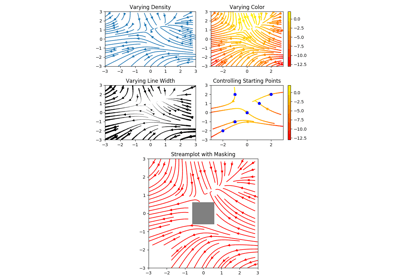/Matplotlib 3.0

# matplotlib.pyplot.streamplot

`matplotlib.pyplot.streamplot(x, y, u, v, density=1, linewidth=None, color=None, cmap=None, norm=None, arrowsize=1, arrowstyle='-|>', minlength=0.1, transform=None, zorder=None, start_points=None, maxlength=4.0, integration_direction='both', *, data=None)` [source]

Draw streamlines of a vector flow.

`x, y : 1d arrays`
an evenly spaced grid.
`u, v : 2d arrays`
x and y-velocities. Number of rows should match length of y, and the number of columns should match x.
`density : float or 2-tuple`
Controls the closeness of streamlines. When `density = 1`, the domain is divided into a 30x30 grid---density linearly scales this grid. Each cell in the grid can have, at most, one traversing streamline. For different densities in each direction, use [density_x, density_y].
`linewidth : numeric or 2d array`
vary linewidth when given a 2d array with the same shape as velocities.
`color : matplotlib color code, or 2d array`
Streamline color. When given an array with the same shape as velocities, color values are converted to colors using cmap.
`cmap : Colormap`
Colormap used to plot streamlines and arrows. Only necessary when using an array input for color.
`norm : Normalize`
Normalize object used to scale luminance data to 0, 1. If None, stretch (min, max) to (0, 1). Only necessary when color is an array.
`arrowsize : float`
Factor scale arrow size.
`arrowstyle : str`
Arrow style specification. See `FancyArrowPatch`.
`minlength : float`
Minimum length of streamline in axes coordinates.
start_points: Nx2 array
Coordinates of starting points for the streamlines. In data coordinates, the same as the `x` and `y` arrays.
`zorder : int`
any number
`maxlength : float`
Maximum length of streamline in axes coordinates.
`integration_direction : ['forward', 'backward', 'both']`
Integrate the streamline in forward, backward or both directions.

Returns:

`stream_container : StreamplotSet`

Container object with attributes

This container will probably change in the future to allow changes to the colormap, alpha, etc. for both lines and arrows, but these changes should be backward compatible.

## Examples using `matplotlib.pyplot.streamplot`Streamplot